# Two thirds

Two thirds of the unknown number is 8/7. What is 7/3 of this number?

a =  4

### Step-by-step explanation: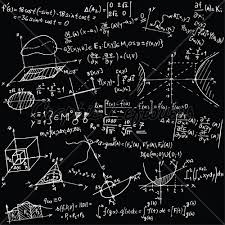Did you find an error or inaccuracy? Feel free to write us. Thank you!Tips to related online calculators
Need help to calculate sum, simplify or multiply fractions? Try our fraction calculator.
Do you have a linear equation or system of equations and looking for its solution? Or do you have a quadratic equation?

## Related math problems and questions:

• FractionsThree-quarters of an unknown number is 4/5. What is 5/6 of this unknown number?
• Unknown number 716% of the unknown number is by 21 less than unknown number itself. Determine the natural unknown number.
• Unknown numberIf I reduced the sum of the numbers 70 and the unknown number three times, I would get 100. what is the unknown number?
• Two thirdsFind two-thirds of the number equal to two-thirds of 99
• Even / odd numbers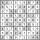a / Using variable n write two consecutive odd numbers. b / the sum of three consecutive odd numbers is 333. What are this numbers?
• Unknown number 24f we add 20, we get 50% of its triple. What is this unknown number?
• Find unknown 2Find unknown denominator: 2/3 -5/? = 1/4
• Marriage sttusIn our city, there are 3/5 of the women married to 2/3 of the men. Find what part of the population is free.
• Unknown number 17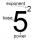Milada said, I am thinking of a number such that I evaluate expression x1/3, the value of the expression would be 5. Which number Milada thinking?
• Algebra problemThis is algebra. Let n represent an unknown number. 1. Eight more than the number n 2. Three times the number n 3. The product of the number n and eight 4. Three less than the number n 5. Three decreased by the number n
• Unknown numberFind an unknown number of which 1/5 is 40 greater than one-tenth of that number.
• Unknown number 5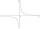Daniel thinks an integer. When he change this number at a ratio of 2:5, he got the number 2.8. Determine what number think Daniel.
• TranslateTranslate the following mathematical statement into an algebraic expression or equation. 1. Nine less that the quotient of a number and 3 2. The ratio of 3 and the sum of 4 and an unknown number 3. Eighty is the product of 4 and g 4. The sum of x and 18 i
• Unknown number 23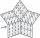Find 2/3 of unknown number, which is two thirds of the 99.
• Unknown number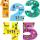Determine the unknown number, which double of its fourth square is equal the fifth its square.
• Unknown mixed numberFind the number that is smaller than 5 5/12 by as much as 2 2/13 is smaller than 6 1/6
• Unknown xIf we add to unknown number 21, then divide by 6 and then subtract 51, we get back an unknown number. What is this unknown number?